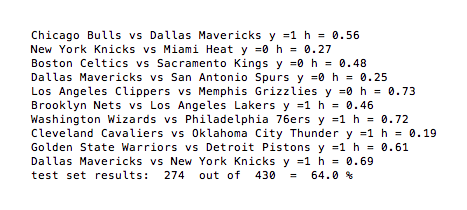The first algorithm:  As mentioned in the prior post, we decided to initially train only on historical win-loss data triples of the form (home team, away team, y), where the Boolean y equals one if the home team won, zero otherwise.  For prediction, we use logistic classification:  We attempt to identify which teams team $\alpha$ would likely beat, were they to play them at home.  In order to accomplish this task, our logistic model has at its disposal a set of variable features characterizing each team:  a home feature vector $\textbf{H}_{\alpha}$ and an away feature vector $\textbf{A}_{\alpha}$, each of length 10.  The model predicts a home team $\alpha$ win over away team $\beta$ probability of $h = 1/[1 + \exp(- \textbf{H}_{\alpha} \cdot \textbf{A}_{\beta})]$ $\in [0,1]$.  In training, the model is initially fed random feature vectors, which are then relaxed to minimize the logistic cost function, $J \equiv$$– \sum_{i = 1}^{m} y_i \log h_i$$+ (1-y_i) \log (1 – h_i)$, where the sum is over all training examples.  The cost function $J$ heavily penalizes large mismatch between the actual outcome $y$ and the predicted outcome $h$ for any training example — we also added to this a suppression term that prevents over-fitting.